#Function Repository Resource:

# FactorGraph

Get a graph representation for the factorization of an integer

Contributed by: Emmanuel Garcés
 ResourceFunction["FactorGraph"][n] gives a graph representing the factorization of the integer n. ResourceFunction["FactorGraph"][n,pos] gives the graph for n with the largest factor with position pos that can be "Inside" or "Outside".

## Details and Options

Let m and n be distinct integers such that m divides n. Then the vertices of a polygon with n vertices can be connected to a polygon with m vertices from m/n equally spaced vertices on the first polygon. This procedure can be applied repeatedly to the prime factors of a number in order to get visualizations of the factorizations of the numbers.
The vertices of a polygon with vertices can be connected to a polygon with vertices from equally spaced vertices on the first polygon. This procedure can be applied repeatedly to the prime factors of a number in order to get visualizations of the factorizations of the numbers.

## Examples

### Basic Examples

Get the rules representing the graph:

 In:=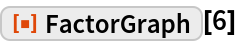Out=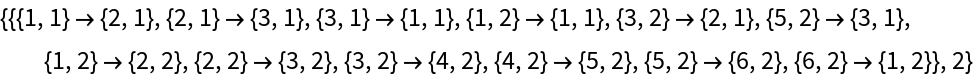In:=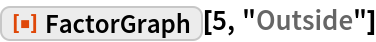Out=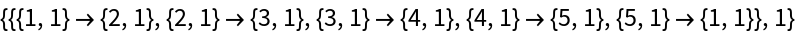Get the graph:

 In:=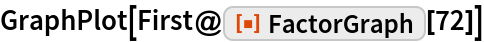Out=Factor graphs from a list of the first 10 integers:

 In:=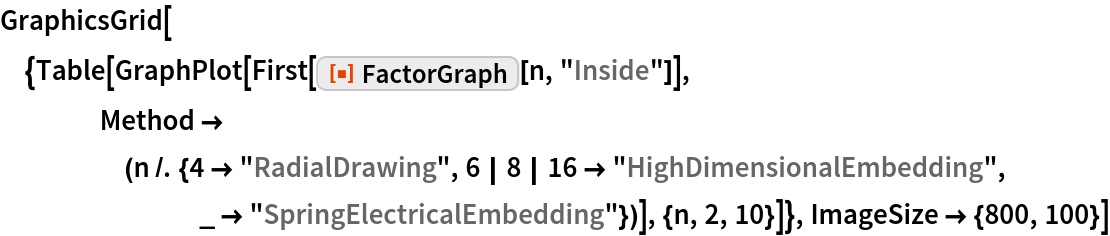Out=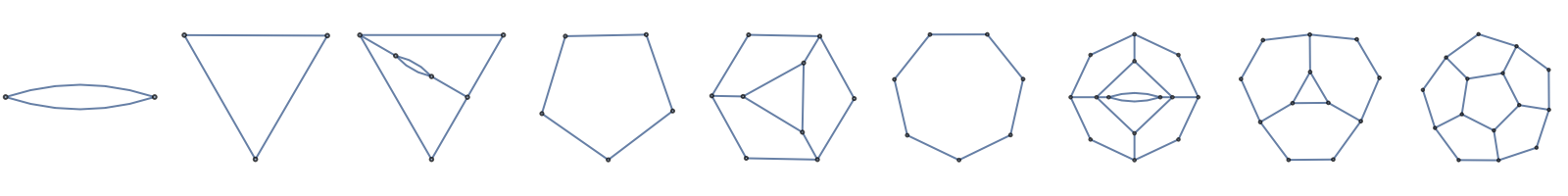With a different method:

 In:=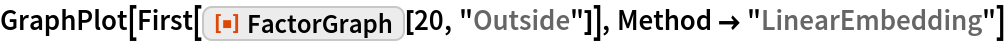Out=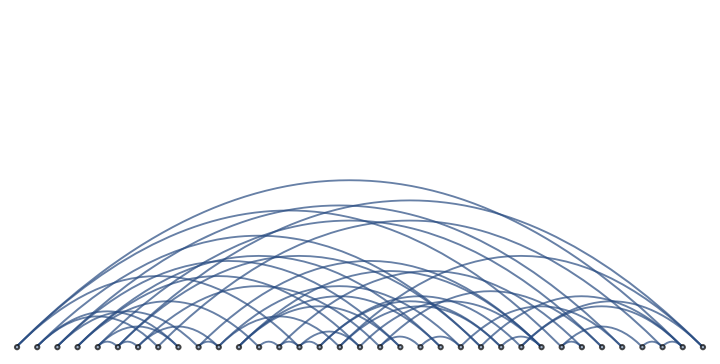For a larger integer:

 In:=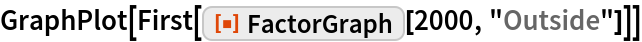Out=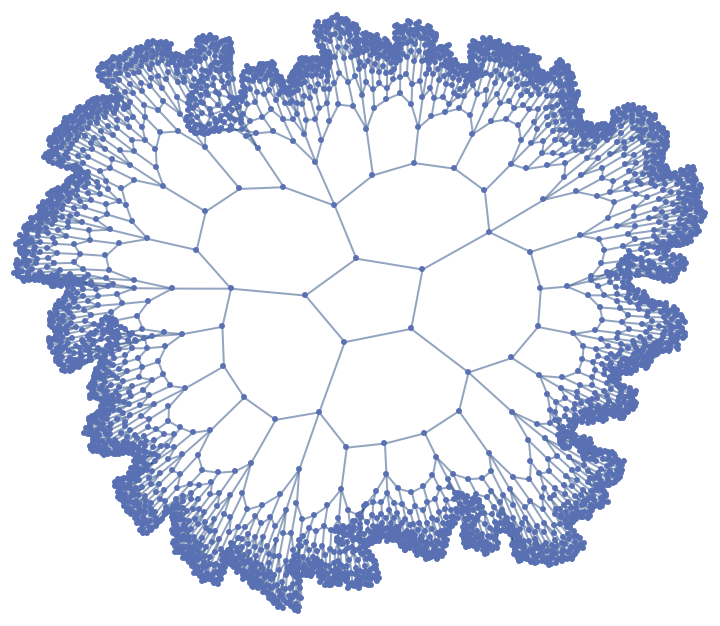## Requirements

Wolfram Language 11.3 (March 2018) or above